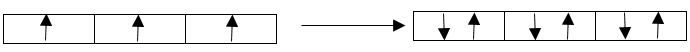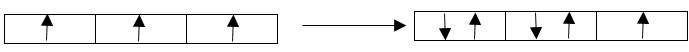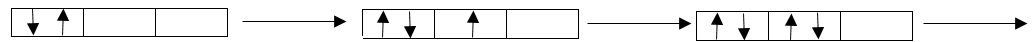QuestionAnswers

# Explain Hund’s rule with an example.

Hint: Hund’s rule is related to the filling of electrons in the s, p, d, and f orbitals in a particular order.

Hund’s rule which is also known as the Hund’s rule of maximum multiplicity states that in the case of filling the orbitals with electrons of a multi-electron atom in its ground state, the sub-level of every orbital must occupy must be singly occupied before the double occupancy starts. All the electrons in the single occupancy must have the same spin for the maximization of the total spin.
Let us understand this rule in a detailed manner.
The electrons are filled in the s, p, d, and f orbitals of an atom. Each orbital has a specific capacity. The capacities of the orbitals are given below:
s orbital – 2 electrons
p orbital – 6 electrons
d orbital – 10 electrons
f orbitals – 14 electrons
Again, in each of the orbitals there are sub-levels that can contain a maximum of 2 electrons. So, there are 1 sub-level for s, 3 sub-levels for p, 5 sub-levels for d, and 7 sub-levels for f.
When the electrons are filled in these orbitals, each of the sub-level is filled first with single electrons in the same spin. After filling all the sub-levels, the electrons are filled for the double occupancy in each sub-level in the opposite spins. For example, for the p orbital, there are 3 sub-levels. So, 3 electrons will be filled in the same spin and the other 3 electrons will be filled one by one in the same-sublevels in the opposite spin.The direction of arrows represents the spin of the electrons and each box are the sublevels of the p orbital.

Let us take the example of fluorine. The electronic configuration of fluorine (F) is ${ 1s }^{ 2 }{ 2s }^{ 2 }{ 2p }^{ 5 }$. Let us understand the filling of the 2p orbital in this case. There are 5 electrons which are to be filled in 3 sub-levels of 2p orbitals. So, the first 3 electrons will be filled in the 3 sub-levels in the same spin and then the rest 2 electrons will be filled in the first two sub-level for double occupancy in the opposite spins leaving the last sub-level with single occupancy.This is the correct way of filling electrons according to the Hund’s rule.This is the incorrect way of filling the electrons and it disobeys Hund’s rule.

Note: Don’t confuse Hund’s rule with Aufbau’s principle. Aufbau’s principle deals with the energy levels of the orbitals. It governs the filling of electrons in different orbitals according to their energy levels while Hund’s rule governs the filling of electrons in an orbital in a particular order.